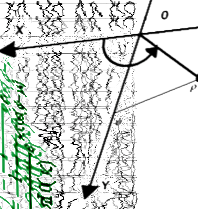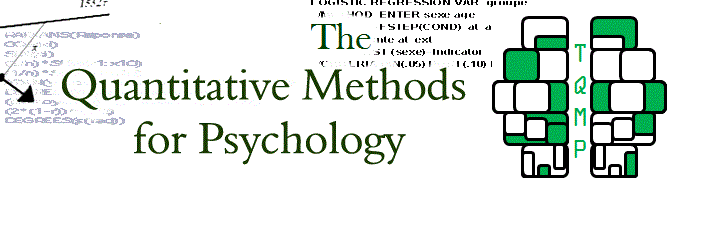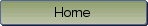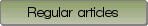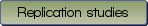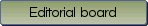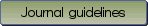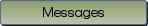An extension of within-subject confidence intervals to models with crossed random effects

Bibliographic information: BibTEX format RIS format XML format APA style
Cited references information: BibTEX format APA style

Politzer-Ahles, Stephen
75-94
Keywords: confidence intervals , repeated measures , linear mixed effects , within-subjects confidence intervals , crossed random effects
(no sample data)   (Appendix)

A common problem in displaying within-subject data is that of how to show confidence intervals that accurately reflect the pattern of significant differences between conditions. The Cousineau-Morey method \parencite {c05,m08} is a widely used solution to this issue; however, this method only applies to experimental designs with only one repeated-measures factor (e.g., subjects). Many experimental designs in fields such as psycholinguistics and social psychology use crossed random effect designs where, e.g., there are repeated measures both for subjects and stimuli. For such designs, extant methods for showing within-subject intervals would require first aggregating over stimuli, and thus such intervals might be a less accurate reflection of the statistical significance patterns if the data are actually analyzed using a method that takes both random effects into account (e.g., linear mixed-effects models). The present paper proposes an extension of the method described above to address this problem; the proposal is to scale the data using a mixed-effects model, rather than using the means from each subject, and then calculate confidence intervals from the data scaled thusly. Analysis of a sample of crossed random effect datasets reveals that intervals calculated using this method give a slightly more accurate reflection of the pattern of statistical significance in the between-condition differences.

Be informed of the upcoming issues with RSS feed:RSS